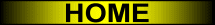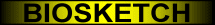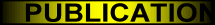Object Localization Based on Directional Information:
Case of 2D Vector Data

ABSTRACT
 If you were told that some object A was perfectly (or somewhat, or not at all) in some direction d (e.g., west, above-right) of some reference object B, where in space would you look for A? Cognitive experiments suggest that you would mentally build a spatial template. Using essentially angular deviation, you would partition the space into regions where the relationship “in direction d of B” holds (to various extents) and regions where it does not hold. You would then be able to locate the objects for which the relationship holds best, and find A. Spatial templates, therefore, represent directional spatial relationships to reference objects (e.g., “east of the post office”). Note that other names can also be found in the literature (e.g., fuzzy landscape, applicability structure, potential field). There exists a very simple and yet cognitively plausible way to mathematically model a spatial template without sacrificing the geometry of the reference object (i.e., the object is not approximated through its centroid or minimum bounding rectangle). In case of 2D raster data, exact calculation of the model can easily be achieved but is computationally expensive, and tractable approximation algorithms were proposed. In case of 2D vector data, exact calculation of the model is not conceivable. In previous work, we introduced the concept of the F-template. We discussed the case of 2D raster data and designed, based on this concept, an efficient approximation algorithm for spatial template computation. The algorithm is faster, gives better results, and is more flexible than its competitors. Here, comparable advances are presented in the case of 2D vector data. These advances are of particular interest for spatial query processing in Geographic Information Systems.